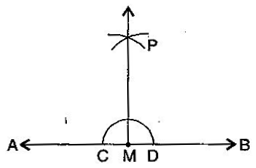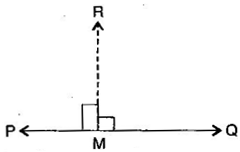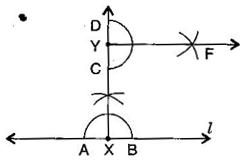1. /
2. CBSE
3. /
4. Class 06
5. /
6. Mathematics
7. /
8. NCERT Solutions for Class...

# NCERT Solutions for Class 6 Maths Exercise 14.4

NCERT solutions for Maths Practical geometry## NCERT Solutions for Class 6 Maths Practical geometry

###### Question 1.Draw any line segment {tex}\overline {{\text{AB}}} .{/tex} Mark any point M on it. Through M, draw a perpendicular to {tex}\overline {{\text{AB}}} .{/tex} (Use ruler and compasses)(i) With M as centre and a convenient radius, draw an arc intersecting the line AB at two points C and B.

(ii) With C and D as centres and a radius greater than MC, draw two arcs, which cut each other at P.

(iii) Join PM. Then PM is perpendicular to AB through the point M.

NCERT Solutions for Class 6 Maths Exercise 14.4

###### Question 2.Draw any line segment {tex}\overline {{\text{PQ}}} .{/tex} Take any point R not on it. Through R, draw a perpendicular to {tex}\overline {{\text{PQ}}} .{/tex} (Use ruler and set-square)(i) Place a set-square on {tex}\overleftrightarrow {{\text{PQ}}}{/tex} such that one arm of its right angle aligns along {tex}\overleftrightarrow {{\text{PQ}}}{/tex}.

(ii) Place a ruler along the edge opposite to the right angle of the set-square.

(iii) Hold the ruler fixed. Slide the set square along the ruler till the point R touches the other arm of the set square.

(iv) Join RM along the edge through R meeting {tex}\overleftrightarrow {{\text{PQ}}}{/tex} at M. Then RM {tex} \bot {/tex} PQ.

NCERT Solutions for Class 6 Maths Exercise 14.4

###### Question 3.Draw a line {tex}l{/tex} and a point X on it. Through X, draw a line segment {tex}\overline {{\text{XY}}} {/tex} perpendicular to {tex}l.{/tex}

Now draw a perpendicular to {tex}\overline {{\text{XY}}} {/tex} to Y. (use ruler and compasses)(i) Draw a line {tex}’l'{/tex} and take point X on it.

(ii) With X as centre and a convenient radius, draw an arc intersecting the line {tex}’l'{/tex} at two points A and B.

(iii) With A and B as centres and a radius greater than XA, draw two arcs, which cut each other at C.

(iv) Join AC and produce it to Y. Then XY is perpendicular to {tex}’l'{/tex}.

(v) With D as centre and a convenient radius, draw an arc intersecting XY at two points C and D.

(vi) With C and D as centres and radius greater than YD, draw two arcs which cut each other at F.

(vii) Join YF, then YF is perpendicular to XY at Y.

## NCERT Solutions for Class 6 Maths Exercise 14.4

NCERT Solutions Class 6 Maths PDF (Download) Free from myCBSEguide app and myCBSEguide website. Ncert solution class 6 Maths includes text book solutions from Class 6 Maths Book . NCERT Solutions for CBSE Class 6 Maths have total 14 chapters. 6 Maths NCERT Solutions in PDF for free Download on our website. Ncert Maths class 6 solutions PDF and Maths ncert class 6 PDF solutions with latest modifications and as per the latest CBSE syllabus are only available in myCBSEguide.

## CBSE app for Students

To download NCERT Solutions for Class 6 Maths, Social Science Computer Science, Home Science, Hindi English, Maths Science do check myCBSEguide app or website. myCBSEguide provides sample papers with solution, test papers for chapter-wise practice, NCERT solutions, NCERT Exemplar solutions, quick revision notes for ready reference, CBSE guess papers and CBSE important question papers. Sample Paper all are made available through the best app for CBSE students and myCBSEguide website.Test Generator

Create question paper PDF and online tests with your own name & logo in minutes.myCBSEguide

Question Bank, Mock Tests, Exam Papers, NCERT Solutions, Sample Papers, Notes

### 2 thoughts on “NCERT Solutions for Class 6 Maths Exercise 14.4”

1. Good !

2. My hard work become easy of any subject